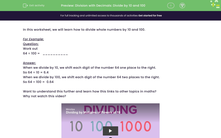# Division with Decimals: Divide by 10 and 100

In this worksheet, students practise dividing whole numbers by 10 and 100.Key stage:  KS 2

Curriculum topic:   Number: Fractions and Decimals

Curriculum subtopic:   Divide by 10 and 100

Difficulty level:#### Worksheet Overview

In this worksheet, we will learn how to divide whole numbers by 10 and 100.

For Example:

Question:

Work out

64 ÷ 100 =   __________

When we divide by 10, we shift each digit of the number 64 one place to the right.

So 64 ÷ 10 = 6.4

When we divide by 100, we shift each digit of the number 64 two places to the right.

So 64 ÷ 100 =  0.64

Want to understand this further and learn how this links to other topics in maths?
Why not watch this video?

### What is EdPlace?

We're your National Curriculum aligned online education content provider helping each child succeed in English, maths and science from year 1 to GCSE. With an EdPlace account you’ll be able to track and measure progress, helping each child achieve their best. We build confidence and attainment by personalising each child’s learning at a level that suits them.

Get started